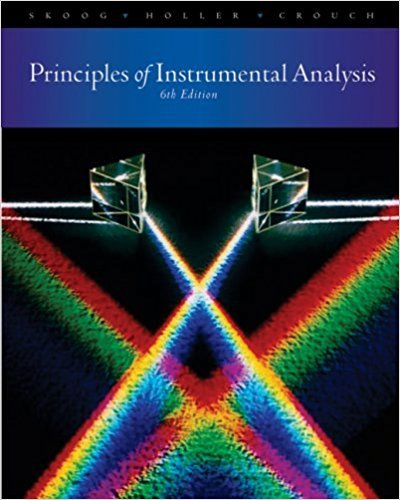×

×

Textbooks / Chemistry / Principles of Instrumental Analysis 6

# Principles of Instrumental Analysis 6th Edition - Solutions by Chapter## Full solutions for Principles of Instrumental Analysis | 6th Edition

ISBN: 9780495012016Principles of Instrumental Analysis | 6th Edition - Solutions by Chapter

Solutions by Chapter
4 5 0 250 Reviews
##### ISBN: 9780495012016

Principles of Instrumental Analysis was written by and is associated to the ISBN: 9780495012016. This textbook survival guide was created for the textbook: Principles of Instrumental Analysis , edition: 6. The full step-by-step solution to problem in Principles of Instrumental Analysis were answered by , our top Chemistry solution expert on 03/02/18, 06:21PM. Since problems from 34 chapters in Principles of Instrumental Analysis have been answered, more than 49826 students have viewed full step-by-step answer. This expansive textbook survival guide covers the following chapters: 34.

Key Chemistry Terms and definitions covered in this textbook
• alkyl group

A group that is formed by removing a hydrogen atom from an alkane. (Section 25.3)

• Anion

An atom or group of atoms bearing a negative charge.

• autoionization

The process whereby water spontaneously forms low concentrations of H+1aq2 and OH-1aq2 ions by proton transfer from one water molecule to another. (Section 16.3)

• band theory.

Delocalized electrons move freely through “bands” formed by overlapping molecular orbitals. (21.3)

• bond cleavage

The breaking of a bond, either homolytically or heterolytically. bond dissociation energy (Sect. 6.1): The energy required to achieve homolytic bond cleavage (generating radicals).

• Bonding molecular orbital

A molecular orbital in which electrons have a lower energy than they would in isolated atomic orbitals

• Brønsted-Lowry base

A proton acceptor

• Dalton’s law of partial pressures.

The total pressure of a mixture of gases is just the sum of the pressures that each gas would exert if it were present alone. (5.6)

• Deshielding

The term used to express the concept of less shielding in NMR

• dipole moment (m)

The amount of partial charge (d ) on either end of a dipole multiplied by the distance of separation (d): m=d × d

• dispersion forces

Intermolecular forces resulting from attractions between induced dipoles. Also called London dispersion forces. (Section 11.2)

• equilibrium-constant expression

The expression that describes the relationship among the concentrations (or partial pressures) of the substances present in a system at equilibrium. The numerator is obtained by multiplying the concentrations of the substances on the product side of the equation, each raised to a power equal to its coefficient in the chemical equation. The denominator similarly contains the concentrations of the substances on the reactant side of the equation. (Section 15.2)

• hemiacetal

A compound containing a hydroxyl group (OH) and an alkoxy group (OR) connected to the same carbon atom.

• Molecular ion (M1)

The radical cation formed by removal of a single electron from a parent molecule in a mass spectrometer.

• nuclear binding energy

The energy required to decompose an atomic nucleus into its component protons and neutrons. (Section 21.6)

• nuclear disintegration series

A series of nuclear reactions that begins with an unstable nucleus and terminates with a stable one; also called a radioactive series. (Section 21.2)

• polarization

For light, the orientation of the electric field.

• reaction mechanism

A series of intermediates and curved arrows that show howthe reaction occurs in terms of the motion of electrons.

• solvolysis

A substitution reaction in which the solvent functions as the nucleophile.

• Unsaturated hydrocarbon

A hydrocarbon containing one or more carbon-carbon double or triple bonds. The three classes of unsaturated hydrocarbons are alkenes, alkynes, and arenes